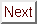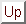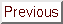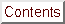Next: Extrinsic Influences on Up: Upper Critical Field Previous: Procedure for Obtaining

# Experimental Results: Angular Dependence of the Upper Critical Field

Two types of data were obtained from the critical field experiments on C4KHgx: Hc2 versus the angle theta at constant temperature, and Hc2 versus temperature at constant angle theta. These two varieties of curves correspond to different cross-sections of the three-dimensional phase boundary, Hc2(theta, T), which separates the superconducting and normal phases.

Hc2(theta) curves taken at about 0.44 K for two C4KHg GIC's are plotted in Figure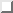. Figurea) shows data taken by Iye on a specimen with Tc = 0.73 K, along with results from an MIT sample with a Tc of 0.95 K. Figureb) shows results from two MIT samples with Tc's of 1.53 and 1.54 K. One prominent feature of the MIT data is the rather large scatter in the critical field values near theta = 90° ( vecH _|_ ^c). This scatter is attributed to error in reading the angular orientation during the experiments. (See Sectionfor an estimate of the measurement errors.) The scatter in Iye's data is much smaller, probably due to a more reliable alignment method, perhaps including a servo-controlled gearing system for rotating the sample. The papers of Iye et al. do not mention what method of sample rotation was employed.Figure: Critical field Hc2 as a function of the angle theta for 4 C4KHg GIC's at T 0.4 K. Fits (dotted lines) were calculated using Equation. a) Data for an MIT C4KHg sample with Tc = 0.95 K (circ) and also for a Tc = 0.73 K sample (bullet) from Ref. . For Tc = 0.95 K sample, 1/epsilon = 10.0 and Hc2(0°) = 24 Oe with a residual cal R = 0.29. For data of Ref. , 1/epsilon = 11.3, Hc2(0°) = 26 Oe, and cal R = 0.090. b) Data for two C4KHg samples with Tc 1.5 K. (circ), Tc = 1.53 K with 1/epsilon = 10.2, Hc2(0°) = 46 Oe, and cal R = 0.73; bullet, Tc = 1.54 K with 1/epsilon = 9.5, Hc2(0°) = 47 Oe, and cal R = 1.18.

The dotted lines in Figureare fits to the equation:where theta is the angle defined in Figurea) and epsilon is the critical field anisotropy parameter of Morris, Coleman and Bhandari,, defined by:The origin of the Hc2(theta) formula[175,127] and the physical interpretation of the epsilon parameter are discussed in more detail in Sectionsand. The residual cal R parameter referred to in Figureis here defined by:where the errors are estimated as:and nu is the number of free parameters == (the number of data points) - (the number of parameters used in the fit plus one). This definition of the residual is similar to that of the reduced chi2 in standard books on statistical analysis of data. However, in the absence of any knowledge of the actual magnitude of the errors in the critical field measurements, the residual parameter should be thought of merely as a convenient figure-of-merit for intercomparison of various fits, rather than as an absolute measure of the appropriateness of Eqn..

Qualitatively, Equationdescribes the experimental points well. Quick comparison of the fits with the points shows that the fit is better for the data of the lower- Tc samples in Figurea) than for the higher- Tc ones in Figureb). While the data points in a) deviate almost randomly from the fit, the data in b) are systematically higher than the fits in the angular region around theta = 0°. One can attempt to improve the situation by raising the parameter Hc2(0°), but since there are only two parameters to vary, the net result is inevitably to worsen agreement in the wings or near the peak, resulting in about the same value of cal R. The situation appears to be similar for some of the data taken by Iye and Tanuma on other superconducting GIC's. For example, Iye and Tanuma's C8RbHg data in Ref.  showed systematic deviations from the fits to Eqn.. Several reasons come to mind as explanations for the systematic deviations seen here; some of these factors are due to extrinsic experimental influences, but others are intrinsic to the physics of layered superconductors, as discussed below.Next: Extrinsic Influences on Up: Upper Critical Field Previous: Procedure for Obtaining

alchaiken@gmail.com (Alison Chaiken)
Wed Oct 11 22:59:57 PDT 1995